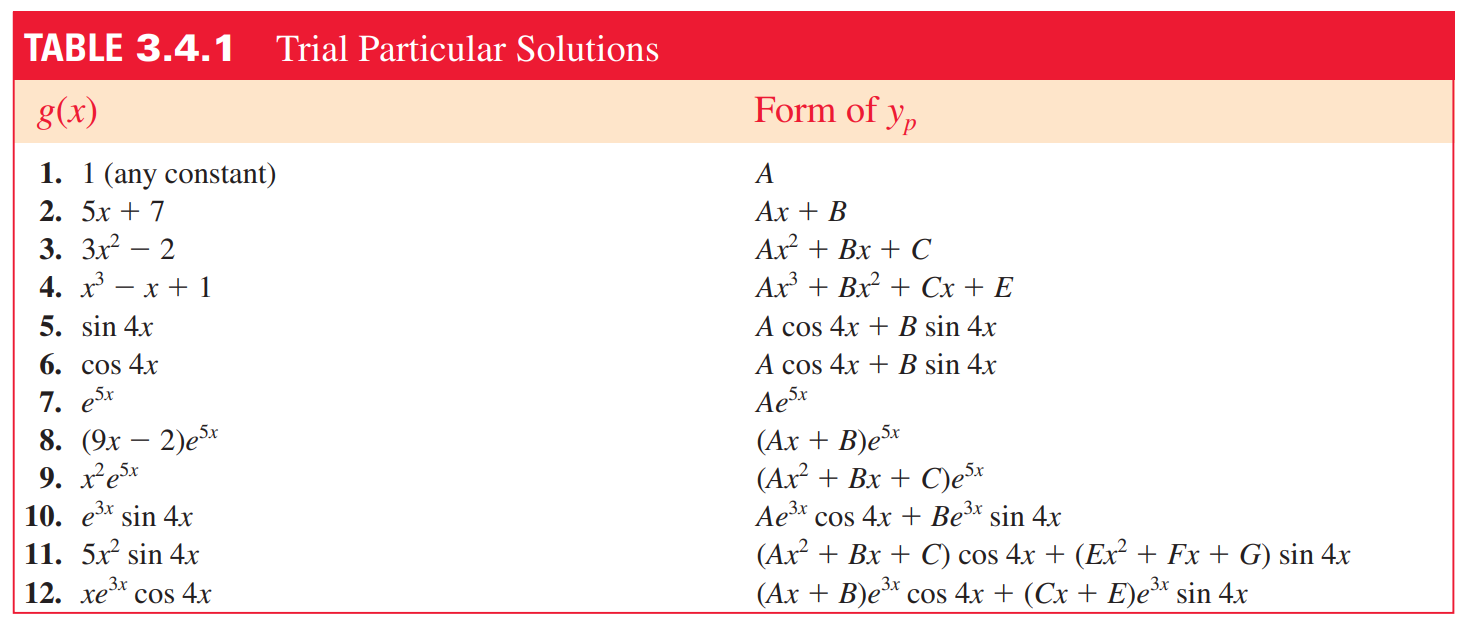# Prerequisites

To better understand the content of this post, it is recommended to know the following:

# Introduction to the Method of Undetermined Coefficients

The method of undetermined coefficients is one of the methods used to solve nonhomogeneous differential equations.

It is generally known as a reliable method for solving differential equations with constant coefficients.

For example, let’s consider the following differential equation (which is the same as equation (9) in The meaning of nonhomogeneous differential equations):

$x''-4x'+3x=t$

We know that the solution $x_p(t)$ that satisfies the nonhomogeneous differential equation can be expressed as follows:

$x_p''-4x_p'+3x_p=t$

The idea behind the method of undetermined coefficients is that the derivative and linear combination of the particular solution will be similar to the particular solution itself. In other words, the idea is based on the observation that differentiating a polynomial function results in another polynomial function, and differentiating an exponential function results in the same exponential function.

Therefore, it is reasonable to assume that the particular solution of the differential equation in equation (1) can be expressed as:

$x_p(t)=At+B$

# Undetermined Coefficients Table

For non-homogeneous terms such as $t$ in equation (1), if they are expressed as polynomials, trigonometric functions, or exponential functions, we can assume a particular solution by choosing undetermined coefficients as shown below and attempt to solve the equation.Figure 1. Forms of particular solutions that can be applied using the undetermined coefficients method.
Image source: Advance Engineering Mathematics, Dennis G. Zill, 6th ed., Jones & Bartlett Learning

It may be noticed that the form of $x_p$ is not so diverse.

In other words, the undetermined coefficients method can only be applied to non-homogeneous terms that are polynomials, sine functions, cosine functions, exponential functions, or linear combinations of these. For other non-homogeneous terms, we need to use the method of variation of parameters.

# Example Problems

## Example Problem 1

Let’s solve a simple example using the undetermined coefficients method.

For example, we can assume the particular solution of equation (1) as

$At+B$

and try to solve the problem.

As we saw in Solution of 2nd Order Linear Differential Equations (2), the general solution of a non-homogeneous differential equation is the sum of its homogeneous solution and particular solution.

The homogeneous solution of equation (1) can be obtained as follows.

The form of homogeneous differential equation (1) is as follows.

$x''-4x'+3x = 0$

Assuming $x=e^{\lambda t}$ and substituting it into the equation, we get,

$\lambda^2e^{\lambda t}-4\lambda e^{\lambda t}+3e^{\lambda t} = 0$

If we group the equation with $e^{\lambda t}$,

$e^{\lambda t}(\lambda^2-4\lambda+3) = 0$

Since $e^{\lambda t}$ is always positive,

$\lambda^2-4\lambda+3 = 0$

and $\lambda$ is

$\lambda = 1 \quad \text{ or } \quad 3$

Therefore, the homogeneous solution is

$x_h = c_1e^t+c_2e^{3t}$

Since we can consider the sum of the homogeneous solution and the particular solution as the general solution, our general solution will be of the following form.

$x(t) = c_1e^t + c_2e^{3t}+At+B$

Meanwhile, since the particular solution must also satisfy the differential equation of the original equation (1),

$x_p' = A$ $x_p'' = 0$

By using these relationships, we can substitute into the original equation (1),

$Equation (1)\Rightarrow -4A+3(At+B)$ $\Rightarrow 3At-4A+3B=t$

Therefore, $3A = 1$ and $-4A+3B = 0$, so

$A=1/3, \quad B = 4/9$

Thus, the general solution of the equation (1) is

$x(t) = c_1e^t + c_2e^{3t}+\frac{1}{3}t+\frac{4}{9}$

## Example problem 2

Find the particular solution of the following differential equation:

$x''-x'+x = 2\sin(3t)$

Although we can assume the particular solution to have the form of $A\sin(3t)$ when looking at this problem, we can also consider that when differentiating $\sin(3t)$ multiple times, we will obtain not only $\sin(3t)$ but also $\cos(3t)$.

Therefore, we can think of the particular solution in the following form:

$x_p=A\cos(3t)+B\sin(3t)$

Now, if we differentiate $x_p$ and substitute it into the original differential equation, we can obtain the following result:

$x_p'' -x_p'+x_p = (-8A-3B)\cos(3t)+(3A-8B)\sin(3t)=2\sin(3t)$

Therefore,

$-8A-3B=0$ $3A-8B = 2$

Thus,

$A=\frac{6}{73},B =-\frac{16}{73}$

Therefore, the particular solution is

$x_p(t)=\frac{6}{73}\cos(3t)-\frac{16}{73}\sin(3t)$

## Example Problem 3

Find the particular solution of the following differential equation:

$x''-5x'+4x=8e^{t}$

This problem is a bit unique, as assuming the particular solution as $Ae^{t}$ as it is in the right-hand side will not solve the problem.

If we substitute it into the original equation, we get:

$Ae^{t}-5Ae^t+4Ae^t=8e^{t}$

This results in a strange result such as $0=e^{8t}$, which indicates that the problem cannot be solved by the traditional method of undetermined coefficients.

The reason why the problem cannot be solved this way is that one of the basis solutions of the homogeneous differential equation is $e^t$.

Let’s take the basis solution $te^t$, which is linearly independent from $e^t$, as the basis solution for the particular solution, similar to what we saw in the reduction of order.

$x_p=Ate^t$

Thus,

$x_p'=Ae^t+Ate^t$

and

$x_p''=Ae^t+Ae^t+Ate^t=2Ae^t+Ate^t$

We can substitute it into the original differential equation, which results in:

$x_p''-5x_p'+4x_p=-3Ae^t=8e^t$

Therefore,

$x_p=-\frac{8}{3}te^t$

is the particular solution.